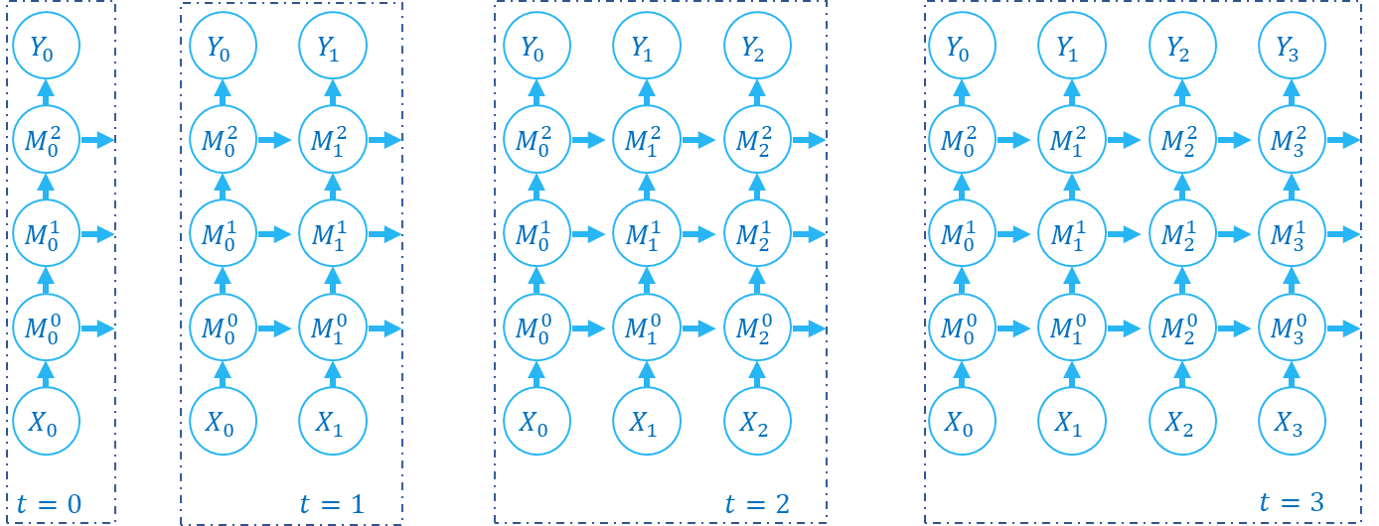Propagation Pattern

Authors: fangwei123456

Single-Step and Multi-Step

Most modules in SpikingJelly (except for spikingjelly.clock_driven.rnn), e.g., spikingjelly.clock_driven.layer.Dropout, don’t have a MultiStep prefix. These modules’ forward functions define a single-step forward:

Input $$X_{t}$$, output $$Y_{t}$$

If a module has a MultiStep prefix, e.g., spikingjelly.clock_driven.layer.MultiStepDropout, then this module’s forward function defines the multi-step forward:

Input $$X_{t}, t=0,1,...,T-1$$, output $$Y_{t}, t=0,1,...,T-1$$

A single-step module can be easily packaged as a multi-step module. For example, we can use spikingjelly.clock_driven.layer.MultiStepContainer, which contains the origin module as a sub-module and implements the loop in time-steps in its forward function:

class MultiStepContainer(nn.Sequential):
def __init__(self, *args):
super().__init__(*args)

def forward(self, x_seq: torch.Tensor):
"""
:param x_seq: shape=[T, batch_size, ...]
:type x_seq: torch.Tensor
:return: y_seq, shape=[T, batch_size, ...]
:rtype: torch.Tensor
"""
y_seq = []
for t in range(x_seq.shape):
y_seq.append(super().forward(x_seq[t]))

for t in range(y_seq.__len__()):
y_seq[t] = y_seq[t].unsqueeze(0)

Let us use spikingjelly.clock_driven.layer.MultiStepContainer to implement a multi-step IF neuron:

from spikingjelly.clock_driven import neuron, layer, functional
import torch

neuron_num = 4
T = 8
if_node = neuron.IFNode()
x = torch.rand([T, neuron_num]) * 2
for t in range(T):
print(f'if_node output spikes at t={t}', if_node(x[t]))
functional.reset_net(if_node)

ms_if_node = layer.MultiStepContainer(if_node)
print("multi step if_node output spikes\n", ms_if_node(x))
functional.reset_net(ms_if_node)

The outputs are:

if_node output spikes at t=0 tensor([1., 1., 1., 0.])
if_node output spikes at t=1 tensor([0., 0., 0., 1.])
if_node output spikes at t=2 tensor([1., 1., 1., 1.])
if_node output spikes at t=3 tensor([0., 0., 1., 0.])
if_node output spikes at t=4 tensor([1., 1., 1., 1.])
if_node output spikes at t=5 tensor([1., 0., 0., 0.])
if_node output spikes at t=6 tensor([1., 0., 1., 1.])
if_node output spikes at t=7 tensor([1., 1., 1., 0.])
multi step if_node output spikes
tensor([[1., 1., 1., 0.],
[0., 0., 0., 1.],
[1., 1., 1., 1.],
[0., 0., 1., 0.],
[1., 1., 1., 1.],
[1., 0., 0., 0.],
[1., 0., 1., 1.],
[1., 1., 1., 0.]])

We can find that the single-step module and the multi-step module have the identical outputs.

Step-by-step and Layer-by-Layer

In the previous tutorials and examples, we run the SNNs step-by-step, e.g.,:

if_node = neuron.IFNode()
x = torch.rand([T, neuron_num]) * 2
for t in range(T):
print(f'if_node output spikes at t={t}', if_node(x[t]))

step-by-step means that during the forward propagation, we firstly calculate the SNN’s outputs $$Y_{0}$$ at $$t=0$$, then we calculate the SNN’s outputs $$Y_{1}$$ at $$t=1$$,…, and we can get the outputs at all time-steps $$Y_{t}, t=0,1,...,T-1$$. The followed code is a step-by-step example (we suppose M0, M1, M2 are single-step modules):

net = nn.Sequential(M0, M1, M2)

for t in range(T):
Y[t] = net(X[t])

The computation graph of forward propagation is built as followed:The forward propagation of SNN and RNN is along both spatial domain and temporal domain. step-by-step calculates states of the whole network step by step. We can also use an another order, which is layer-by-layer. layer-by-layer calculates states layer-by-layer. The followed code is a layer-by-layer example (we suppose M0, M1, M2 are multi-step modules):

net = nn.Sequential(M0, M1, M2)

Y = net(X)

The computation graph of forward propagation is built as followed:The layer-by-layer method is widely used in RNN and SNN, e.g., Low-activity supervised convolutional spiking neural networks applied to speech commands recognition calculates outputs of each layer to implement a temporal convolution. Their codes are availble at https://github.com/romainzimmer/s2net.

The difference between step-by-step and layer-by-layer is the order of traverse the computation graph. The computed results of both methods are exactly same. However, step-by-step has more degree of parallelism. When a layer is stateless, e.g., torch.nn.Linear, the step-by-step method may calculate as:

for t in range(T):
y[t] = fc(x[t])  # x.shape=[T, batch_size, in_features]

The layer-by-layer method can calculate parallelly:

y = fc(x)  # x.shape=[T, batch_size, in_features]

For a stateless layer, we can concatenate inputs shape=[T, batch_size, ...] at time dimension as shape=[T * batch_size, ...] to avoid loop in time-steps. spikingjelly.clock_driven.layer.SeqToANNContainer has provided such a function in its forward. We can directly use this module:

T = 16
batch_size = 8
x = torch.rand([T, batch_size, 4])
fc = SeqToANNContainer(nn.Linear(4, 2), nn.Linear(2, 3))
print(fc(x).shape)

The outputs are

torch.Size([16, 8, 3])

The outputs have shape=[T, batch_size, ...] and can be directly fed to the next layer.

Wrap Forward Propagation

After we use SeqToANNContainer to wrap stateless ANN’s layers, the .keys() of network’s state_dict will change because we introduce an external wrapper. Here is an example:

net_step_by_step = nn.Sequential(
nn.BatchNorm2d(16),
neuron.IFNode()
)

net_layer_by_layer = nn.Sequential(
layer.SeqToANNContainer(
nn.BatchNorm2d(16),
),
neuron.MultiStepIFNode()
)

print('net_step_by_step.state_dict:', net_step_by_step.state_dict().keys())
print('net_layer_by_layer.state_dict:', net_layer_by_layer.state_dict().keys())

The outputs are:

net_step_by_step.state_dict: odict_keys(['0.weight', '1.weight', '1.bias', '1.running_mean', '1.running_var', '1.num_batches_tracked'])
net_layer_by_layer.state_dict: odict_keys(['0.0.weight', '0.1.weight', '0.1.bias', '0.1.running_mean', '0.1.running_var', '0.1.num_batches_tracked'])

We can find that keys have been changed, which causes some trouble to load model’s weights. For example, if we want to build a multi-step Spiking ResNet-18 (spikingjelly.clock_driven.model.spiking_resnet.spiking_resnet18), and we want to load the pre-train model’s weights from ANN. If the network is built by SeqToANNContainer, it wil be not able to load weights from ANN because keys of state_dict are different. To avoid such problems, we can wrap forward propagation, rather than wrap layers. Here is an example:

class NetStepByStep(nn.Module):
def __init__(self):
super().__init__()
self.conv = nn.Conv2d(3, 16, kernel_size=3, padding=1, bias=False)
self.bn = nn.BatchNorm2d(16)
self.sn = neuron.IFNode()

def forward(self, x):
# x.shape = [N, C, H, W]
x = self.conv(x)
x = self.bn(x)
x = self.sn(x)
return x

class NetLayerByLayer1(NetStepByStep):

def forward(self, x_seq):
# x_seq.shape = [T, N, C, H, W]
x_seq = functional.seq_to_ann_forward(x_seq, [self.conv, self.bn])
x_seq = functional.multi_step_forward(x_seq, self.sn)
return x_seq

class NetLayerByLayer2(NetStepByStep):
def __init__(self):
super().__init__()

# replace single-step neuron to multi-step neuron
del self.sn
self.sn = neuron.MultiStepIFNode()

def forward(self, x_seq):
# x_seq.shape = [T, N, C, H, W]
x_seq = functional.seq_to_ann_forward(x_seq, [self.conv, self.bn])
x_seq = self.sn(x_seq)
return x_seq

state_dict.keys() of NetStepByStep, NetLayerByLayer1, NetLayerByLayer2 are identical, and they can load model weights from each others.